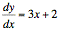Te Kete Ipurangi
Communities
Schools

### Te Kete Ipurangi user options:

Achievement objectives

What has changed:

# Achievement objective M7-10

In a range of meaningful contexts, students will be engaged in thinking mathematically and statistically. They will solve problems and model situations that require them to:

• apply differentiation and anti-differentiation techniques to polynomials.

## Indicators

• Develops the rules for differentiation of polynomials using understanding of gradient function and technology (graphics calculator or computer).
• Solves differentiation problems involving:
• rates of change
• finding points where gradient has a particular value
• finding the equation of the tangent to a curve at a point
• finding local maxima/minima of a function
• kinematics.
• Develops rules for anti-differentiation of polynomials using understanding of gradient function and technology (graphics calculator or computer).
• Solves anti-differentation problems involving:
• finding a family of curves with a given gradient
• finding the constant of anti-differentiation
• simple polynomial differential equations (not including separation of variables).
• Make links with graphs M7-9.
• See key mathematical ideas on NZmaths.

## Progression

M7-10 links from NA6-4, GM6-3 and to M8-11, M8-12.

## Possible context elaborations

• If the gradient function of a curve isand the curve passes through (1, 5), find the equation of the curve.
• Optimisation, for example, finding maximum area of a rectangular paddock for a given constraint on the length, cost functions, optimising volume.
• Box construction: Constructing a box with restricted resource and requirements.
• Fencing problems
• Maximising the volume of a rectangular box
• Packaging investigation: Maximising product in a package.
• Tennis ball drop: Finding the height using anti-differentiation.

## Assessment for qualifications

NCEA achievement standards at level 1, 2 and 3 have been aligned to the New Zealand Curriculum. Please ensure that you are using the correct version of the standards by going to the NZQA website.

The following achievement standard(s) could assess learning outcomes from this AO:

Last updated September 17, 2018